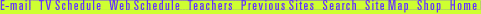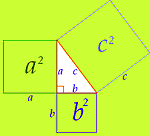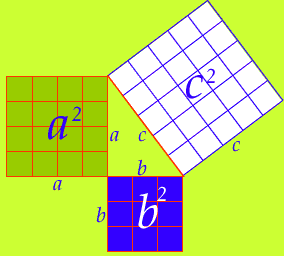## Demonstrate the Pythagorean Theorem

Think of each side of a right triangle as also being a side of a square that's attached to the triangle. The area of a square is any side multiplied by itself. (For example, a x a = a2).To show that a2 + b2 = c2, follow these steps:
1. Click here to get a right triangle on grid paper that you can print. You'll also need scissors, and a ruler.

2. Cut out the triangle.

3. Make three squares with sides that are equal to each side of the triangle. Begin with side a. Measure the length of side a. On the blank piece of grid paper, draw a square with sides that are the same length as side a. Label this square a2. Repeat these steps to create squares for sides b and c. (If you don't have a ruler, just use the triangle as a guide; trace the length of one side, and then draw three more sides of the same length to make a square.)

4. Cut out the squares. Place each square next to the corresponding sides of the triangle.5. Now show that a2 + b2 = c2. Place the squares made from sides a and b on top of square c. You will have to cut one of the squares to get a perfect fit.

Congratulations! You've shown that the Pythagorean theorem works! But how can you use it?

Andrew Wiles | Math's Hidden Woman | Pythagorean Puzzle
Resources | Guide | Transcript | Proof Home